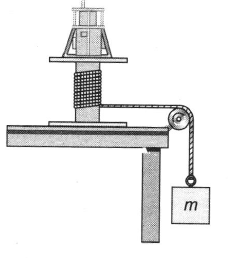Figure shows a counterweight of mass m suspended by a cord wound around a spool of radius r, forming part of a turntable supporting the object. The turntable can rotate without friction. When the counterweight is released from rest, it descends through a distance h, acquiring a speed v. The moment of inertia I of the rotating apparatus is

# Figure shows a counterweight of mass m suspended by a cord wound around a spool of radius r, forming part of a turntable supporting the object. The turntable can rotate without friction. When the counterweight is released from rest, it descends through a distance h, acquiring a speed v. The moment of inertia I of the rotating apparatus is1. A

${\mathrm{mr}}^{2}\left(\frac{2\mathrm{gh}}{{\mathrm{v}}^{2}}+1\right)$

2. B

${\mathrm{mr}}^{2}\left(\frac{\mathrm{gh}}{{\mathrm{v}}^{2}}-1\right)$

3. C

${\mathrm{mr}}^{2}\left(\frac{2\mathrm{gh}}{{\mathrm{v}}^{2}}-1\right)$

4. D

${\mathrm{mr}}^{2}\left(\frac{\mathrm{gh}}{{\mathrm{v}}^{2}}+1\right)$

Register to Get Free Mock Test and Study Material

+91

Verify OTP Code (required)

### Solution:

Each point on the cord moves at a linear speed of v = $\mathrm{\omega r}$ where r is the radius of the spool. The energy conservation equation for the counter weight turn table-Earth system is:

Specializing, we have

mgh = $\frac{1}{2}{\mathrm{mv}}^{2}+\frac{1}{2}\mathrm{I}\frac{{\mathrm{v}}^{2}}{{\mathrm{r}}^{2}}$

2mgh -Register to Get Free Mock Test and Study Material

+91

Verify OTP Code (required)